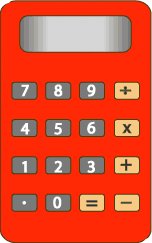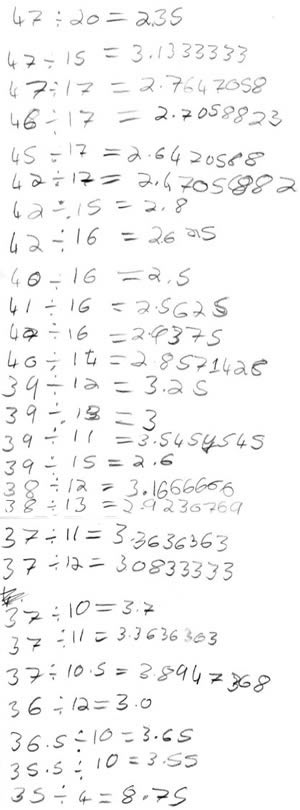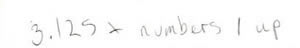#### You may also like### Cubes

How many faces can you see when you arrange these three cubes in different ways?### Pebbles

Place four pebbles on the sand in the form of a square. Keep adding as few pebbles as necessary to double the area. How many extra pebbles are added each time?### Bracelets

Investigate the different shaped bracelets you could make from 18 different spherical beads. How do they compare if you use 24 beads?

# Forgot the Numbers

## Forgot the Numbers

If you are a teacher click here for a version of the problem suitable for classroom use, together with supporting materials. Otherwise, read on ...On my calculator I divided one whole number by another whole number and got the answer $3.125$.

I know that both numbers were less than $50$, but can't remember what they were.

Can you work out what they were?

Once you've had a chance to think about it, click below to see how four different pupils began working on the task.

Here is Gemma and Flo's work:Richard wrote the following:He explained:

"I multiplied $3.125$ by $1$, then I tried multiplying $3.125$ by $2$, then I multiplied $3.125$ by $3$ ..."

Here is the start of Thomas' work:

I first looked at the number $0.125$ and worked out what fraction of $1$ it is. It turned out that it was an eighth.

Can you take each of these starting ideas and develop it into a solution?

### Why do this problem?

This task has the potential to address many aspects of number and calculation, including the inverse relationship between multiplication and division, and the relationship between division and decimal fractions.  The richness of the activity comes in the many different approaches which could be used to solve it and discussion of these different methods is emphasised in these notes.  You may well need to spend a couple of lessons on this activity.

### Possible approach

Pose the problem orally, or project the text onto a screen, without mentioning the examples of how some children started.  Give the class a few minutes to consider, individually, how they might go about tackling the problem, then pair them up and suggest that they talk to their partner about their ideas so far.  Try to stand back and observe, and resist the temptation to make helpful suggestions!

Allow pairs to work on the task so that you feel they have made some progress, but do not worry if they have not completed it or if they report being stuck.  The aim at this stage is for everyone to 'get into' the problem and work hard on trying to solve it, but not necessarily to achieve a final solution.

At a suitable time, hand out this (doc   pdf) to pairs.  Suggest to the class that when they've finished or can't make any further progress, they should look at the sheet showing three approaches used by children working on this task.  Pose the question, "What might each do next? Can you take each of their starting ideas and develop them into a solution?".  You may like pairs to record their work on large sheets of paper, which might be more easily shared with the rest of the class in the plenary.

Allow at least fifteen minutes for a final discussion.  Invite some pairs to explain how the three different methods might be continued.  You may find that some members of the class used completely different approaches when they worked on the task to begin with, so ask them to share their methods too.  You can then facilitate a discussion about the advantages and disadvantages of each.  Which way would they choose to use if they were presented with a similar task in the future? Why?

### Key questions

Can they apply what they have learnt to a similar problem, where the answer is $3.375$, $4.5$, $4.1$?